Z Transform of Convolution Next  |  Prev  |  Up  |  Top  |  Index  |  JOS Index  |  JOS Pubs  |  JOS Home  |  Search

## Z Transform of Convolution

From Eq. (5.5), we have that the output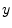from a linear time-invariant filter with input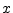and impulse response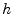is given by the convolution ofand, i.e.,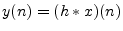(7.3)

where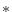'' means convolution as before. Taking the z transform of both sides of Eq. (6.3) and applying the convolution theorem from the preceding section gives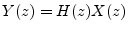(7.4)

where H(z) is the z transform of the filter impulse response. We may divide Eq. (6.4) by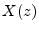to obtain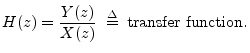This shows that, as a direct result of the convolution theorem, the z transform of an impulse responseis equal to the transfer function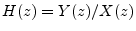of the filter, provided the filter is linear and time invariant.

Next  |  Prev  |  Up  |  Top  |  Index  |  JOS Index  |  JOS Pubs  |  JOS Home  |  Search

[How to cite this work] [Order a printed hardcopy]